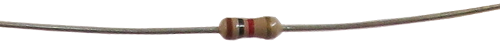Starting Electronics Needs Your Help!
It is that time of the year when we need to pay for web hosting and buy new components and equipment for new tutorials. You can help by making a donation. Contribute to this website by clicking the Donate button. The total will be updated once daily. (You may need to clear your browser cache to see the updates.)Target Amount: \$2000
Amount Raised: \$1579.10

Top Donor: C.C. \$100

X

# Resistor

Created on: 30 July 2012

There are two schematic symbols for a resistor that are used in circuit diagrams as shown below:When beginning electronics, we usually use quarter Watt five percent tolerance resistors (1/4W, 5%). These resistors look like this:## Resistance

Resistors have a resistance that is given in Ohms (symbol Ω), e.g. 470 ohms (or 470Ω). The resistor above has a value of 1 kilo ohms which is written as 1kΩ or just 1k.

## Power

Resistors have a power rating - the above resistor is rated at 1/4W (or 250mW). The higher the power rating a resistor has, the bigger its physical size will be.

## Tolerance

Resistors have a tolerance rating given as a percentage. The above resistor has a tolerance of 5%. This means that its ohm value could be 5% greater or 5% less than its quoted value. The above resistor has a value of 1k (1000 ohms) and a tolerance of 5% - this means that its value could be between 950 ohms and 1050 ohms (1000 - 5% to 1000 + 5%).

The coloured bands that you see on the resistor tell us what its value is in ohms and what its tolerance is. The gold band tells us that its tolerance is 5%.

## Finding the Resistor Value

A resistor's value in ohms is worked out from the first three coloured bands using the table below. Using the resistor above, its bands are brown, black and red. The first colour, brown, has a value of 1. The second colour, black, has a value of 0 (zero). The third colour tells us how many zeros the value has - red = 2 zeros. Putting it all together we get: 1, 0, and two zeros (00) = 1000 ohms or 1k.

More examples:

Resistor colours - Yellow, Violet, Brown = 4, 7, 1 (number of zeros is 1) = 47 and one zero = 470 ohms

Resistor colours - Red, Red, Red = 2, 2, 2 = 22 and 2 zeros = 2200 ohms = 2.2k, usually written as 2k2

Resistor colours - Yellow, Violet, Red = 4, 7, 2 = 4, 7, 00 = 4700 ohms or 4.7k = 4k7

Resistor colours - Brown, Black, Orange = 1, 0, 3 = 1, 0, 000 = 10000 = 10k

Resistor colours - Orange, Orange, Black = 3, 3, 0 (no zeros) = 33 ohms

## Resistor Colour Chart

 Colour Value Black 0 Brown 1 Red 2 Orange 3 Yellow 4 Green 5 Blue 6 Violet 7 Grey 8 White 9